# Reflection In 2D Graphics

• Last Updated : 12 Oct, 2020

Reflection deals with obtaining a mirror image of the 2D object.

If P(x, y) is the point on x-y plane then P’(x’, y’) is the reflection about x-axis given as x’=x ; y’=-y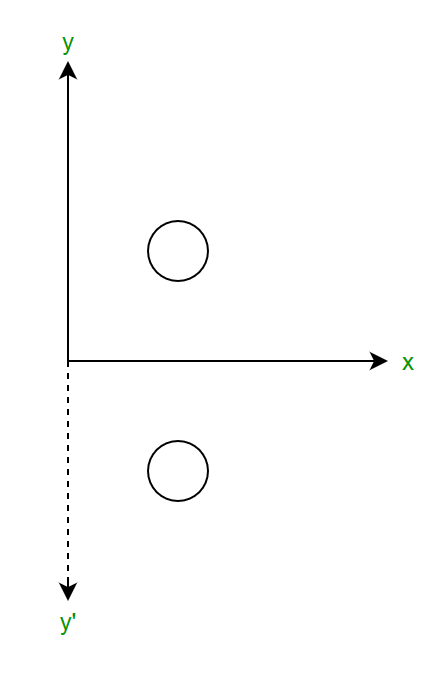Matrix Form:If P(x, y) is the point on x-y plane then P’(x’, y’) is the reflection about y-axis given as x’=-x ; y’=y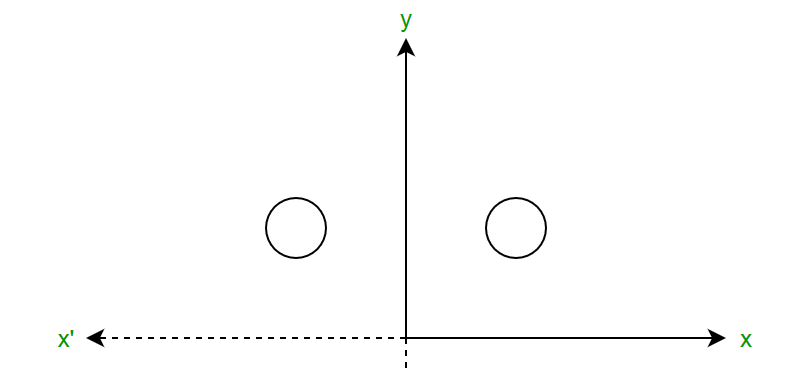Along origin :
If P(x, y) is the point on x-y plane then P’(x’, y’) is the reflection about origin given as x’=-x ; y’=-y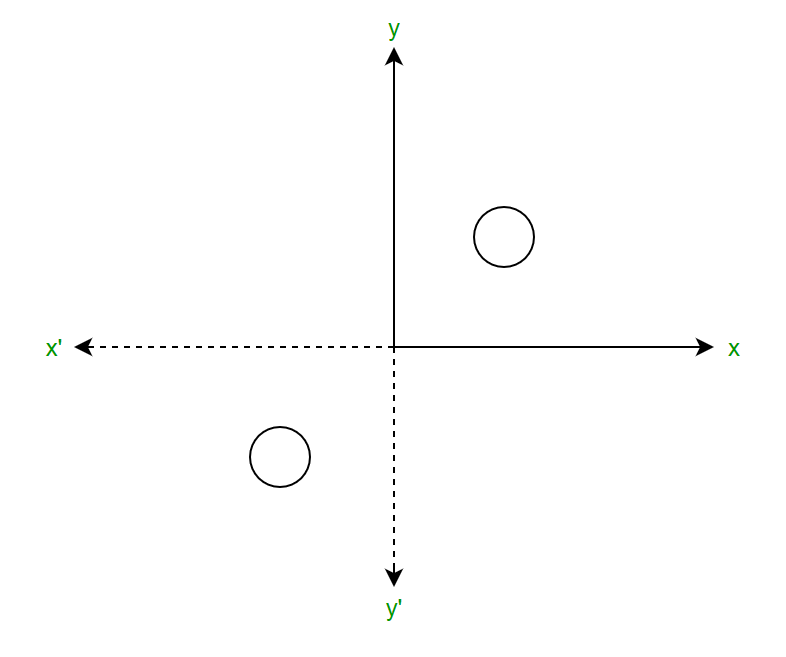About x=y line : To do this move x=y line to any of the axis. In the given diagram the angle of rotation is 45o as the points are plotted as (0, 0), (1, 1), (2, 2), and so on.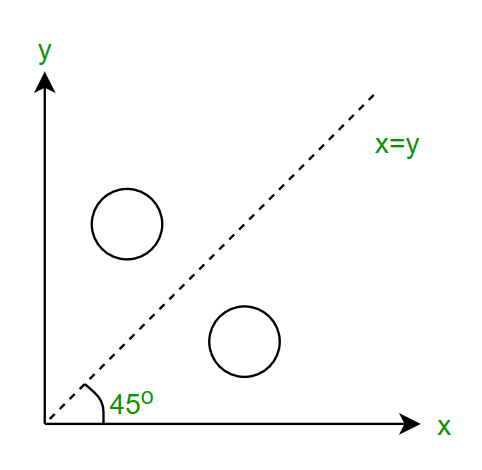Imposing the line clockwise (-45o) imposing it on the x-axis we have,We know,andNow perform reflection along x-axis,Now rotate the line back 45o in an anticlockwise direction,Now if P(x, y) is the point on x-y plane then P’(x’, y’) is the reflection about x=y line given as x’=y ; y’=x
Matrix Form:Problem: A triangle is given with the coordinates p (5 4), q (2 2), r (5 6) we need to reflect it along Y-axis.

Ans: We are given with coordinate p, q, r as shown in figure-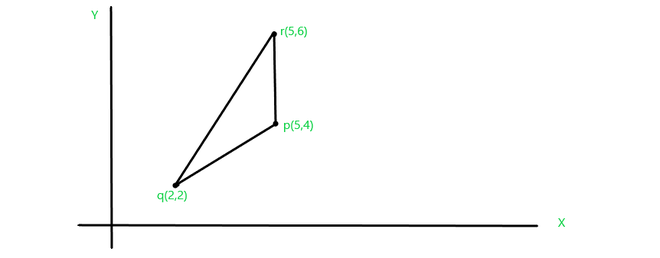Triangle reflection in 2-d graphics

Now, we apply the condition of reflecting a 2-d object along Y-axis:First coordinate p, becomes p’ after reflection:Second coordinate q, becomes q’ after reflection:Third coordinate r of the triangle becomes r’ after reflection:After reflecting the triangle about the Y-axis point p, q, r becomes p’, q’, r’ :

p(5, 4) = p'(-5, 4) , q(2, 2)
= q'(-2, 2) , r(5, 6)
= r'(-5, 6) 

The reflecting object would appear as :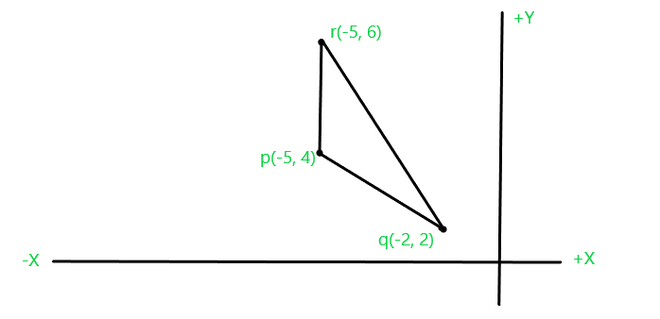My Personal Notes arrow_drop_up